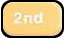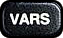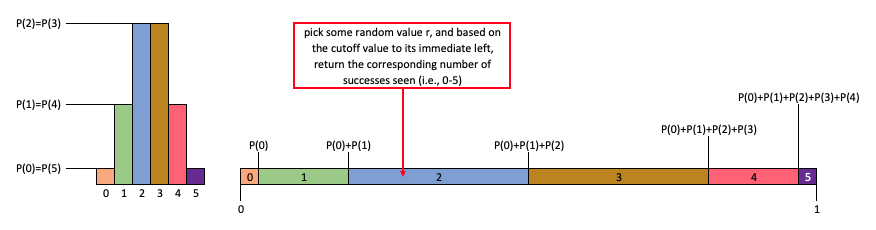## Tech Tips: Binomial Distributions

### Calculating $P(X=x)$ when $X$ follows a Binomial Distribution

Suppose one wishes to find the binomial probability of seeing exactly $k$ successes in $n$ independent trials, where the probability of success on any one trial is $p$ and the probability of failure is $q = 1-p$. That is to say, we seek $$P(k) = ({}_n C_k) p^k q^{n-k}$$ To do this, one should ...

• R: use the function

dbinom(x=k, size=n, prob=p)


As an example, to find the probability that one flips exactly 4 heads in 8 tosses of a fair coin:

> dbinom(x=4, size=8, prob=1/2)
 0.2734375


• Excel: use the function

BINOM.DIST(k, n, p, FALSE)

The last argument for this function, when $FALSE$, indicates that the probability returned should not be cumulative (i.e., it only returns $P(k)$, not $P(0) + P(1) + \cdots + P(k)$).

• TI-83: use the function

binompdf(n,p,k)

This function can be found by making the following menu selections:: binompdf(

### Calculating Cumulative Probabilities when $X$ follows a Binomial Distribution

Suppose one wishes to fine the cumulative binomial probability of seeing $k$ or fewer successes in $n$ independent trials, where the probability of success on any one trial is $p$ and the probability of failure is $q = 1-p$. That is to say, we seek $$P(X \le k) = P(0) + P(1) + P(2) + \cdots + P(k) = \sum_{0 \le i \le k} ({}_n C_i) p^i q^{n-i}$$ To do this, one should ...

• R: use the function

pbinom(k, size = n, prob = p)

As an example, Suppose there are 12 multiple choice questions on a quiz. Each question has five possible answers, and only one of them is correct. One can find the probability of having four or less correct answers if a student attempts to answer every question at random using
> pbinom(4, size=12, prob=1/5)
 0.9274445

It should be noted that this gives you the same answer as the following - just with a lot less typing!
> dbinom(0, size=12, prob=0.2) +
+ dbinom(1, size=12, prob=1/5) +
+ dbinom(2, size=12, prob=1/5) +
+ dbinom(3, size=12, prob=1/5) +
+ dbinom(4, size=12, prob=1/5)
 0.9274445


Importantly, if we instead wanted the probability of the student getting somewhere between $4$ and $8$ (inclusive) questions correct, we can use a difference of two cumulative probabilities, as the below illustrates:

> pbinom(8, size=12, prob=1/5) - pbinom(3, size=12, prob=1/5)
 0.2053689

Be mindful of the 3 in the calculation above. Recall, if we want to calculate $$P(4 \le X \le 8) = P(4) + P(5) + P(6) + P(7) + P(8)$$ this equals the difference $$\require{color}{\color{purple}[P(1) + P(2) + P(3) + P(4) + P(5) + P(6) + P(7) + P(8)]} - {\color{green}[P(1) + P(2) + P(3)]}$$

• Excel: use the function

BINOM.DIST(k, n, p, TRUE)

The last argument for this function, when $TRUE$, indicates the probability returned should be cumulative. That is to say, it gives the sum $P(0) + P(1) + \cdots + P(k)$.

• TI-83: use the function

binomcdf(n,p,k)

This function can be found by making the following menu selections:: binomcdf(

### Simulating Random Variables following Binomial Distributions

To generate $m$ realizations of a random variable that follows a binomial distribution, counting the number of successes seen in $n$ independent trials, where the probability of success on any one trial is $p$, ...
• R: use the function

rbinom(x=m, size=n, prob=p)

Be careful not to confuse the number realizations of your binomial random variable that you are generating (i.e., $m$ in this case) with the number of trials (i.e., given by the parameter "size", here equal to $n$). This is a common source of error.

Each of the two examples below independently simulates $12$ trials where the probability of success in each trial is $1/5$, and returns the number of successes seen. Note, there is a random element to rbinom(), so it can (and does) return different values when you run it at different times.

> rbinom(1,size=12,prob=1/5)
 2

> rbinom(1,size=12,prob=1/5)
 4


If one wants to run this experiment several times, one just alters the first parameter to the function. Below, we run $12$ trials a total of $6$ times, returning the number of successes seen each time.

> rbinom(6,size=12,prob=1/5)
 3 4 5 2 2 2


• Excel: use the function

BINOM.INV(n,p,RAND())

One might wonder why the $RAND()$ function is passed as a parameter to the $BINOM.INV()$ function. The reason has to do with what $BINOM.INV()$ actually does.

To use a concrete example, let us suppose that the context in which we are using this function involves flipping a coin 5 times and counting the number of heads.

We know the probability mass function is given by $P(k)=({}_nC_k)p^kq^{n-k}$, but given that the $k$ values involved are simply $0,1,2,3,4,\textrm{ and } 5$, we can use this formula to construct a table that represents the probability mass function as well. It is shown below.

$$\begin{array}{|l|c|c|c|c|c|c|}\hline k & 0 & 1 & 2 & 3 & 4 & 5\\\hline P(k) & \frac{1}{32} & \frac{5}{32} & \frac{10}{32} & \frac{10}{32} & \frac{5}{32} & \frac{1}{32}\\\hline \end{array}$$ We can see the values of $P(k)$ reach a maximum when $k=2$ or $k=3$ from the table. If we used each $P(k)$ value as the height of a rectangle centered at each $x=k$, we can see the nature of the distribution of $P(k)$ values even better (as seen on the left in the diagram below).

Now, imagine disassembling this "pile" of rectangles, laying each one down -- end to end -- from $x=0$ to $x=1$. Recall that the sum of the $P(k)$ values (i.e., the rectangle lengths) must be exactly $1$, so this is possible to do.What $BINOM.INV()$ does is to take the value of its last parameter and find where on this line of rectangles from $0$ to $1$ it falls. It then uses the cutoff values between rectangles, as calculated by cumulative sums of $P(k)$ values, to locate the cutoff value to its immediate left. The corresponding number of successes seen (a number from 0 to 5) that is paired with this cutoff value is then found and returned by the function.

So by passing $RAND()$ as the last parameter to $BINOM.INV()$, a random position in the "line of rectangles" is chosen. The way in which we constructed these rectangles assures us that the values returned by $BINOM.INV()$ follow the correct binomial probabilities.

In the diagram above, it appears that the random value $r$ picked falls between $P(0)+P(1)$ and $P(0)+P(1)+P(2)$ -- making it correspond to the blue bar associated with 2 successes seen in the 5 trials total. Thus, for this random value $r$, $BINOM.INV()$ would return 2.